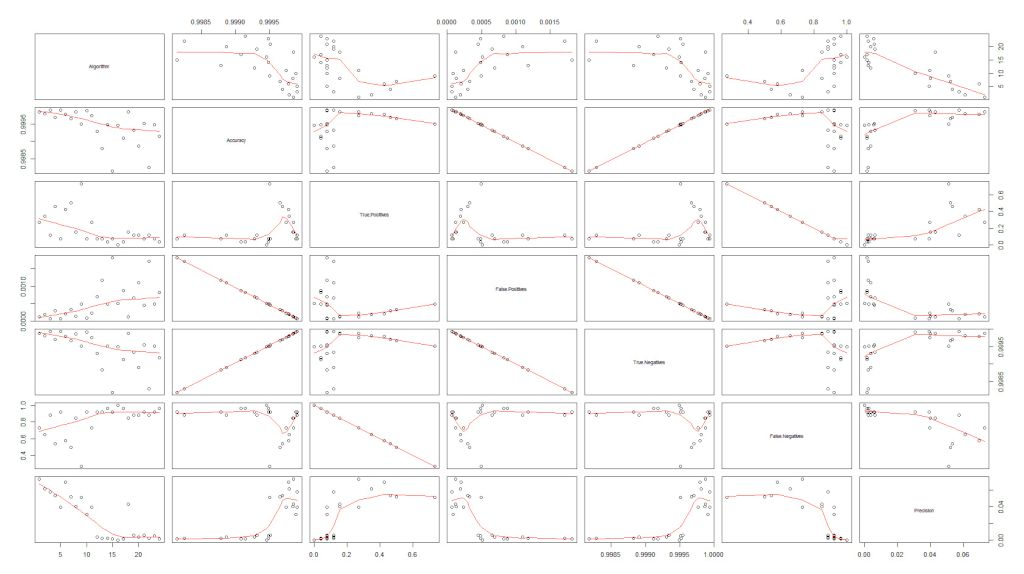📅 March 3, 2012 🕐 15:56 🏷 Thesis (MSc)

As I am starting to gather testing data, I figured it’d be a good time to determine how to measure the performance of the different results of my 24 text representation algorithms. What I want to measure per algorithm: how many keywords are predicted the same as the experts, and how many aren’t. After some research and valuable advice, I came across confusion matrices, which seemed appropriate. For each algorithm I measure the amount of:

 Predicted Negative Positive Actual Negative A B Positive C D

A. True Negatives (excluded by algorithm & excluded by experts)
B. False Positives (included by algorithm & excluded by experts)
C. False Negatives (excluded by algorithm & included by experts)
D. True Positives (included by algorithm & included by experts)

I found this page from the University of Regina explaining confusion matrices, and decided to implement it. My implementation in pseudocode:

```For each text:

fill expertList with expertResults #list of URIs
fill algoList with algorithmResults #list of URIs

algoPOS = algoList #URIs included by algorithm
algoNEG = [item for item in NCIthesaurus if item not in algoPOS] #URIs excluded by algorithm (ontology-algoPOS)
expertPOS = expertList #all URIs included by experts
expertNEG = [item for item in NCIthesaurus if item not in expertPOS] #all URIs excluded by experts (ontology-expertPOS)

A += len(set(algoNEG).intersection(expertNEG)) #True Negatives (number of URIs that overlap in algoNEG and expertNEG)
B += len(set(algoPOS).intersection(expertNEG)) #False Positives (number of URIs that overlap of algoPOS and expertNEG)
C += len(set(algoNEG).intersection(expertPOS)) #False Negatives (number of URIs that overlap in algoNEG and expertPOS)
D += len(set(algoPOS).intersection(expertPOS)) #True Positives (number of URIs that overlap in algoPOS and expertPOS)

matrix.append([[A,B],[C,D]]) #Put numbers in a matrix

```

With this information I can calculate a set of standard terms:
Accuracy (AC), Recall or True Positive Rate (TP), False Positive Rate (FP), True Negative Rate (TN), False Negative Rate (FN), Precision (P).

```  AC = ((A+D) / (A+B+C+D))
TP = ((D) / (C+D))
FP = ((B) / (A+B))
TN = ((A) / (A+B))
FN = ((C) / (C+D))
P = ((D) / (B+D))
```

The only thing with the rates are that proportions are heavily skewed because of the size of the ontology (90.000 URIs), which means the negative cases will always be much more frequent than the positives. This means that accuracy is always around 99.9%, and so is the TN. I still have to figure out exactly what information I want to use and how (visualize?).